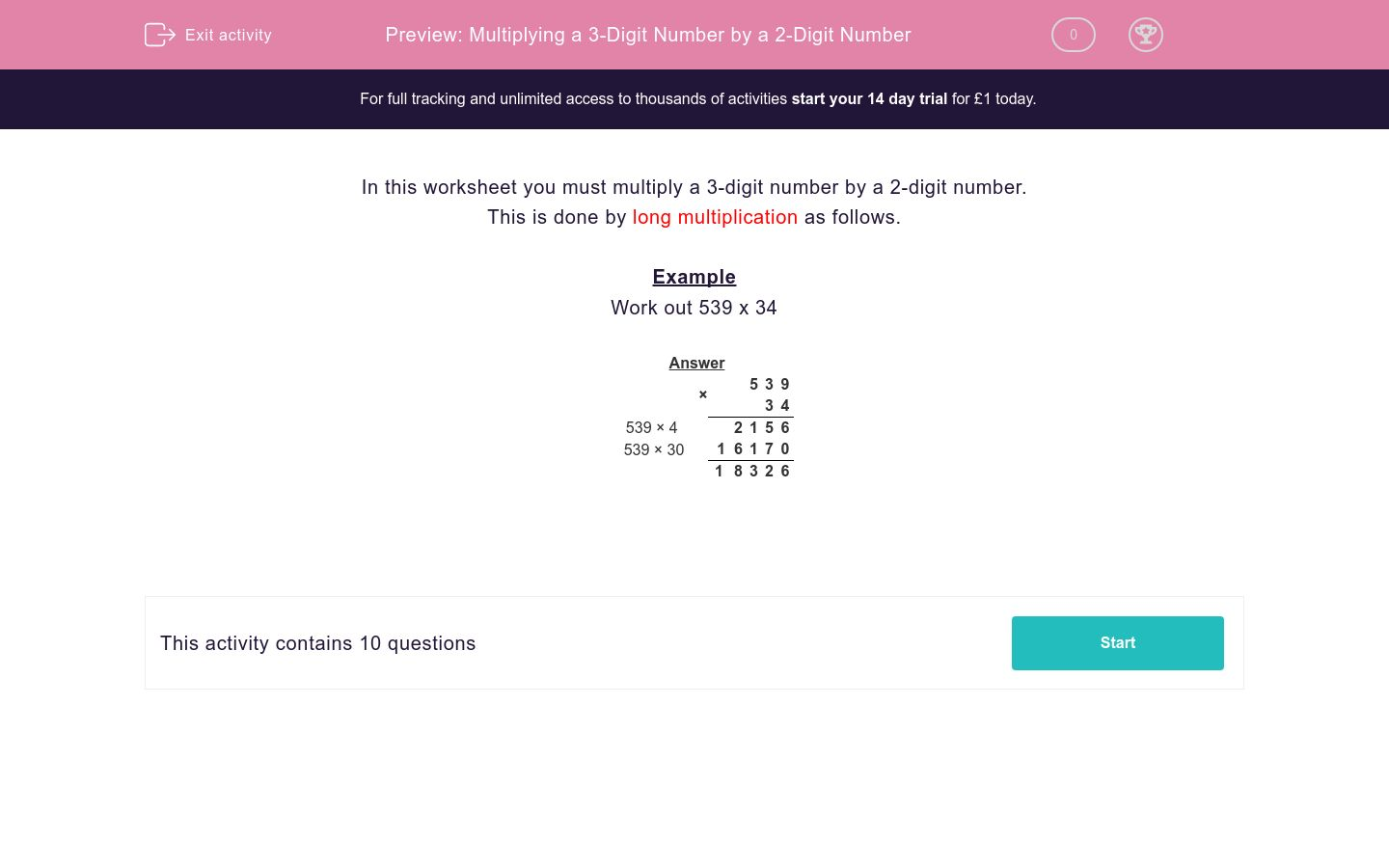# Multiplying a 3-Digit Number by a 2-Digit Number

In this worksheet, students multiply a 3-digit number by a 2-digit number.Key stage:  KS 2

Curriculum topic:   Maths and Numerical Reasoning

Curriculum subtopic:   Mixed Problems

Difficulty level:#### Worksheet Overview

In this worksheet you must multiply a 3-digit number by a 2-digit number.

This is done by long multiplication as follows.

Example

Work out 539 x 34

 × 5 3 9 3 4 539 × 4 2 1 5 6 539 × 30 1 6 1 7 0 1 8 3 2 6

### What is EdPlace?

We're your National Curriculum aligned online education content provider helping each child succeed in English, maths and science from year 1 to GCSE. With an EdPlace account you’ll be able to track and measure progress, helping each child achieve their best. We build confidence and attainment by personalising each child’s learning at a level that suits them.

Get started